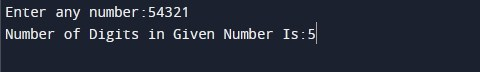Program to Count Number Of Digits In Number

In this tutorial you will learn about the Program to Count Number Of Digits In Number and its application with practical example.

In this tutorial, we will learn to create a C Program to Count Number Of Digits In Number. For example: if the input number is 4321, then the output of the program will be 4. We will show you different method to count number of digits in number:

• Using while loop
• Using logarithmic function
• By creating function to count digits
• Using recursion

Prerequisites

Before starting with this tutorial we assume that you are best aware of the following C programming topics:

• C Data Types
• C Operator
• C If else
• C while loop
• C Function
• C Recursion

Program to Count Number Of Digits In Number using while loop

In this program we will count number of digits In number using while loop.. We have first declared and initialized the required variables. Next, we would prompt user to input a number to count number of digits in it. Later in the program we will count number of digits using while loop. We will then display the number of digits in given number.

Output:-Next, we have declared and initialized a set variables required in the program.

• num = it hold the first integer number value
• count = will hold count of digit in number

In the next statement user will be prompted to enter an integer number which will be assigned to variable ‘num’. Next, we will compute the number of digits in a given number using c while loop. In the program, we use the division(/) operator to obtain individual digits of the number and increase count by 1(count = count + 1). Finally, we will be displaying the number of digits In ‘num’ using printf statement.

Program to Count Number Of Digits In Number using logarithmic function

In this program we will count number of digits In number using logarithmic function log10(). We have first declared and initialized the required variables. Next, we would prompt user to input a number to count number of digits in it. Later in the program we will count number of digits using log10() function. The log10() is the predefined function in math.h. We will then display the number of digits in given number.

Output:-In the above program, we have used log10() function to count number of digits in a given number. The log10() is the predefined function in math.h. The formula to calculate number of digits using log10() function is as following:

Next, we have declared and initialized a set variables required in the program.

• num = it hold the first integer number value
• count = will hold count of digit in number

In the next statement user will be prompted to enter an number which will be assigned to variable ‘num’. Next, we will use log10() function to count number of digits in a given number. Finally, we will be displaying the number of digits In ‘num’ using printf statement.

Program to Count Number Of Digits In Number using function

In this program we will count number of digits In number using c function. We have first declared and initialized the required variables. Next, we would prompt user to input a number to count number of digits in it. Later in the program we will count number of digits using a user defined function. We will then display the number of digits in given number.

Output:-In the above program, we have first defined following function:

digits() :- This function means to accept an integer number and return the number of digits In that number.

Next, we have declared and initialized a set variables required in the program.

• num = it hold the first integer number value
• count = will hold count of digit in number

In the next statement user will be prompted to enter an number which will be assigned to variable ‘num’. Next, we will be calling digits() function to count number of digits In number. Finally, we will be displaying the number of digits In ‘num’ using printf statement.

Program to Count Number Of Digits In Number using recursion

In this program we will count number of digits In number using a recursive function. A function is called recursive when calls itself. We have first defined a recursive function in the program to return number of digits In given number. Next, we have declared and initialized the required variables. We would then prompt user to input a number. Later in the program we have invoked the digits() function to find number of digits in given number. We will then display the number of digits in given number.

Output:-In the above program, we have first defined a function called as digits(). This digits() function will find number of digits in given number. Next, we have declared required variables in the main function.

• num = it holds the input number value
• count = will hold count of digit in number

Next, user prompted to enter a number which assigned to variable ‘num’. Next, we will be calling digits() function to count number of digits In number. Finally, we will display the number of digits In given number.

In this tutorial we have learn about the Program to Count Number Of Digits In Number and its application with practical example. I hope you will like this tutorial.